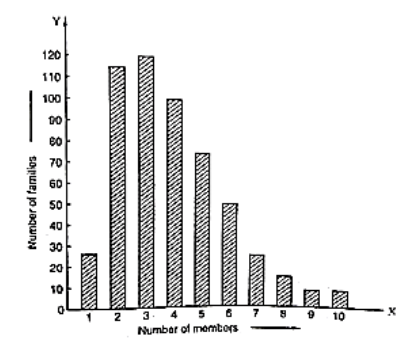# Read the bar graph given in and answer the following questions:`
Question:(i) What information is given by the bar graph?

(ii) What was the crop-production of rice in 1970 – 71?

(iii) What is the difference between the maximum and minimum production of rice?

Solution:

(i) The bar graph represents the production of the rice crop in India in different years.

(ii) According to the height of the 3rd bar from the left, the crop-production of rice in 1970 – 71 is 42.5 lakh tonnes.

(iii) The maximum product of rice is 55 lath tonnes (height of the 4th bar from the left) in the year 1980 – 81 and the minimum product of rice is 22 lath tonnes (height of the 1st bar from the left) in the year 1950 – 51. Hence, the difference between maximum and minimum production of rice (in lath tonnes) is 55 – 22 = 33